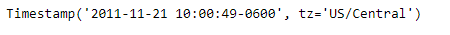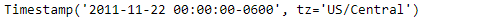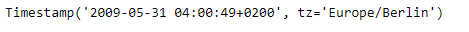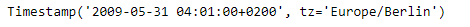GeeksforGeeks App
Open AppBrowser
Continue

# Python | Pandas Timestamp.ceil

Python is a great language for doing data analysis, primarily because of the fantastic ecosystem of data-centric python packages. Pandas is one of those packages and makes importing and analyzing data much easier.

Pandas` Timestamp.ceil()` function return a new Timestamp ceiled to this resolution. The function takes the desired time series frequency as an input.

Syntax : Timestamp.ceil()

Parameters :
freq : a freq string indicating the ceiling resolution

Return : Timestamp

Example #1: Use `Timestamp.ceil()` function to ceil the given Timestamp object to Daily time series frequency.

 `# importing pandas as pd``import` `pandas as pd`` ` `# Create the Timestamp object``ts ``=` `pd.Timestamp(year ``=` `2011``,  month ``=` `11``, day ``=` `21``,``           ``hour ``=` `10``, second ``=` `49``, tz ``=` `'US/Central'``)`` ` `# Print the Timestamp object``print``(ts)`

Output :Now we will use the `Timestamp.ceil()` function to ceil the ts object to Daily frequency.

 `# ceil the given object to daily frequency``ts.ceil(freq ``=``'D'``)`

Output :As we can see in the output, the `Timestamp.ceil()` function has ceiled the time series frequency of the given Timestamp object to the input frequency.

Example #2: Use `Timestamp.ceil()` function to ceil the given Timestamp object to minutely time series frequency.

 `# importing pandas as pd``import` `pandas as pd`` ` `# Create the Timestamp object``ts ``=` `pd.Timestamp(year ``=` `2009``,  month ``=` `5``, day ``=` `31``,``        ``hour ``=` `4``, second ``=` `49``, tz ``=` `'Europe/Berlin'``)`` ` `# Print the Timestamp object``print``(ts)`

Output :Now we will use the `Timestamp.ceil()` function to ceil the ts object to minutely frequency.

 `# ceil the given object to minutely frequency``ts.ceil(freq ``=``'T'``)`

Output :As we can see in the output, the `Timestamp.ceil()` function has ceiled the time series frequency of the given Timestamp object to the input frequency.

My Personal Notes arrow_drop_up